# Benchmarking Modular Mojo🔥 and PyTorch torch.compile() on Mandelbrot function

Quick comparision of Mandelbrot function acceleration using PyTorch 2.0’s torch.compile() and Modular’s Mojo🔥

Last week, Modular - an startup co-founded by Chris Lattner (of LLVM, Swift, MLIR fame), announced a brand new high-performance language called Mojo🔥. Mojo🔥 looks and reads like Python but that’s only on the surface, underneath the familiar Python syntax Mojo uses it’s own JIT and AOT compilation process to accelerate Python code. Although Mojo doesn’t fully support all of Python today, according to Mojo docs, over time Mojo is expected to become a superset of Python.PyTorch's got some Mojo🔥Mojo isn’t open-source yet and can only be accessed from the Mojo playground. So I promptly applied and got access to Mojo Playground a couple of days after the accouncement on May 3rd. First thing I wanted to do was to compare Mojo’s performance to PyTorch. While PyTorch is a popular framework for deep learning, its also a capable replacement for numpy as a high-performance scientific computing library.

And my favorite feature of PyTorch is the new `torch.compile()` API introduced in PyTorch 2.0 that can accelerate arbitrary functions (with limitations) written using the PyTorch API. It takes PyTorch highlevel API, optimizes it and generates C++ or GPU code to improve it’s performance. I’ve discussed `torch.compile()` in great detail in my blog post, and I highly recommend reading it if you want to learn about how PyTorch compiler does operator fusion and CPU/GPU code-generation:

##### How Pytorch 2.0 Accelerates Deep Learning with Operator Fusion and CPU/GPU Code-Generation

In this blog post I want to discuss relative performance between Mojo🔥 and PyTorch, and I picked the Mandelbrot example that Jeremy Howard (or FastAI fame) demoed during the Modular keynote. I reimplemnted Mojo’s Mandelbrot example in PyTorch to compared it’s performance with Mojo. Before I get into the code, here are the results.

Summary: Mojo is fast! and PyTorch is no slouch either!

Language/FrameworkMandelbrot execution
(200 iterations)
System
PyTorch GPU `torch.compile()`~165 μs (micro seconds)Intel Core i7-9700K CPU @ 3.60GHz + NVIDIA Titan V
Mojo CPU~2.6 msMojo Playground: Intel Xeon Platinum 8375C CPU @ 2.90GHz
PyTorch CPU `torch.compile()`~9 msIntel Core i7-9700K CPU @ 3.60GHz
PyTorch GPU~15 msIntel Core i7-9700K CPU @ 3.60GHz + NVIDIA Titan V
PyTorch CPU~50 msIntel Core i7-9700K CPU @ 3.60GHz
PyTorch Apple M2 MPS~70 msMacbook Pro M2 Apple Silicon + 30-Core GPU + 16-Core Neural Engine
Python/numpy~152 msIntel Core i7-9700K CPU @ 3.60GHz

#### Interesting observations

• Mojo is the fastest CPU implementation
• PyTorch GPU with `torch.compile()` generates a fused cuda kernel making it the fastest on GPU
• PyTorch CPU with `torch.compile()` which generates fused C++ code is still faster than PyTorch GPU without compilation

It should come as no surprise that PyTorch generated custom fused kernel for Mandelbrot function running on GPU is indeed faster than Mojo CPU, it’s not even a fair comparision. PyTorch CPU is only slightly slower, but makes up for performance with better usability. Mojo is harder to use and I’m positive the UX will improve over time.

## Benchmarks and caveats

This is not a scientific benchmark test. This is a rather crude, and hacked-together-in-a-day example that should illustrate the performance differences and coding approaches, so take it with a grain of Sodium Chloride.

#### My naive testing methodology:

• I use Jupyter’s native `timeit` with `10` repeats to benchmark and report mean and variance.
• For GPU, I call `torch.cuda.synchronize()` before measurement to ensure that the kernel is fully executed.
• This code example also benchmarks tensors/arrays creation which is in the body of the function, which migh not be a feature that arises in real-world scenarios.
• I don’t measure the compilation time for PyTorch 2.0 and that does take a lot of time to generate loop-unrolled C++ code for CPU and NVPTX for GPU.

#### Hardware differences

Mojo is not open-source (yet), and the only way to run it is on the early access Mojo Playground which has a different configuration compared to my desktop running PyTorch. For the Apple M2 benchmark I also use a MacBook Pro laptop for M2 testing. Suffice to say, this is not a fair comparison. Hardware details:

• PyTorch on Desktop
• CPU: Intel Core i7-9700K CPU @ 3.60GHz with 8 cores
• GPU: NVIDIA Titan V
• PyTorch on Mac
• Apple MacBook Pro with M2 Apple Silicon
• Mojo🔥 on Mojo Playground
• Intel Xeon Platinum 8375C CPU @ 2.90GHz with 32 cores
Update (05/09/23): Chris Lattner pointed out that Mojo doesn’t use all 32 cores on the Mojo Playground. That is mindblowing performance on 4 cores! 🤯

## Why is PyTorch so much faster than Python/Numpy on CPU?

PyTorch is faster than Python/Numpy because the higher level PyTorch API calls highly optimized C++ routines implemented in the ATen library. These routines are eagerly evaluated, which means that each PyTorch API call is executed immediately which adds some function call overhead with every API call. To address this, PyTorch 2.0 introduced a compilation API called `torch.compile()` which takes eager PyTorch code, optimizes it and generates C++ code with OpenMP pragmas for parallelization on CPU or generates GPU code using OpenAI Triton. This is similar to what Numba does for Python code, but PyTorch 2.0’s `torch.compile()` is much more powerful because it can fuse multiple PyTorch API calls into a single kernel, which reduces function call overhead and improves performance.

I’ve discussed this in detail in my PyTorch 2.0 blog post, but here is a screenshot of what the automatically generated fused kernels for C++ for CPU and OpenAI Triton for GPU look like for Mandelbrot function

Autogenerated C++ code for Mandelbrot function with `torch.compile()`
#####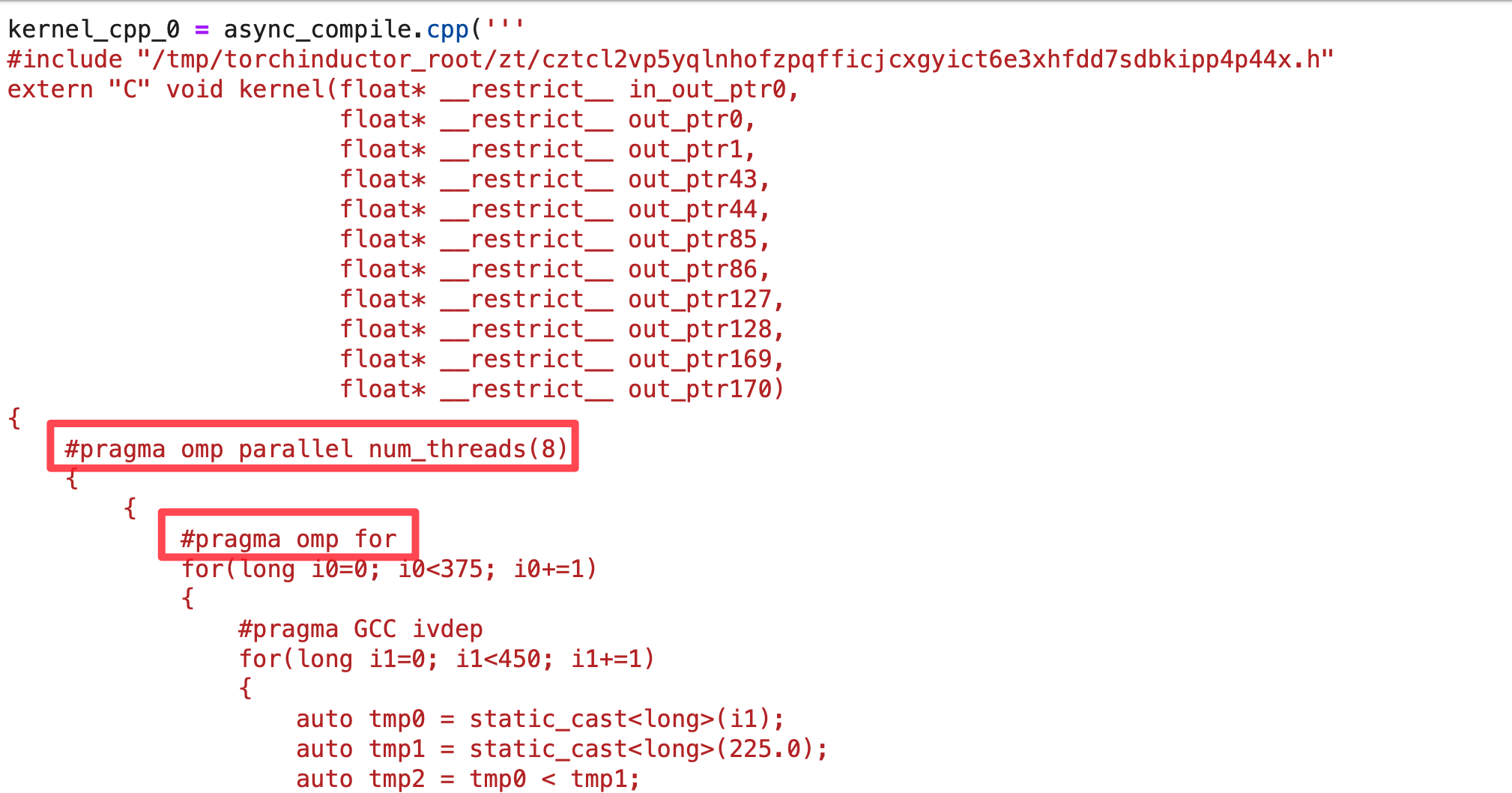Autogenerated OpenAI Triton code for Mandelbrot function with `torch.compile()`

## Baseline: Benchmarking Python/Numpy

Let’s start with a baseline. Here’s the Python/Numpy implementation of Mandelbrot function. I’ve also included a function to plot the Mandelbrot set using matplotlib. We’ll later modify this function to use PyTorch.

``````import numpy as np
import torch
import matplotlib.pyplot as plt
import matplotlib.colors as colors
import time
import warnings

def mandelbrot_numpy(max_iter=200):
# Define the boundaries of the complex plane
xn = 450
yn = 375
xmin = -2.25
xmax = 0.75
ymin = -1.25
ymax = 1.25

# Create the grid of complex numbers
x_values = np.linspace(xmin, xmax, xn, dtype=np.float64)
y_values = np.linspace(ymin, ymax, yn, dtype=np.float64)
rx, iy = np.meshgrid(x_values, y_values, indexing='xy')

x = rx.copy()
y = iy.copy()
for i in range(max_iter):
x_prev = x
y_prev = y
x = x_prev**2 - y_prev**2 + rx
y = 2*x_prev*y_prev + iy
inside = np.sqrt(x**2 + y**2) <= 2

def make_plot_python(m):
xn = 450
yn = 375
dpi = 32
width = 5
height = 5 * yn // xn

fig = plt.figure(1, [width, height], dpi=dpi)
ax = fig.add_axes([0.0, 0.0, 1.0, 1.0], frame_on=False, aspect=1)

light = colors.LightSource(315, 10, 0, 1, 1, 0)

image = light.shade(m, plt.cm.hot, colors.PowerNorm(0.3), blend_mode='hsv', vert_exag=1.5)
plt.imshow(image)
plt.axis("off")
plt.show()
``````

Output: With a baseline established let’s compare the performance of PyTorch and Mojo.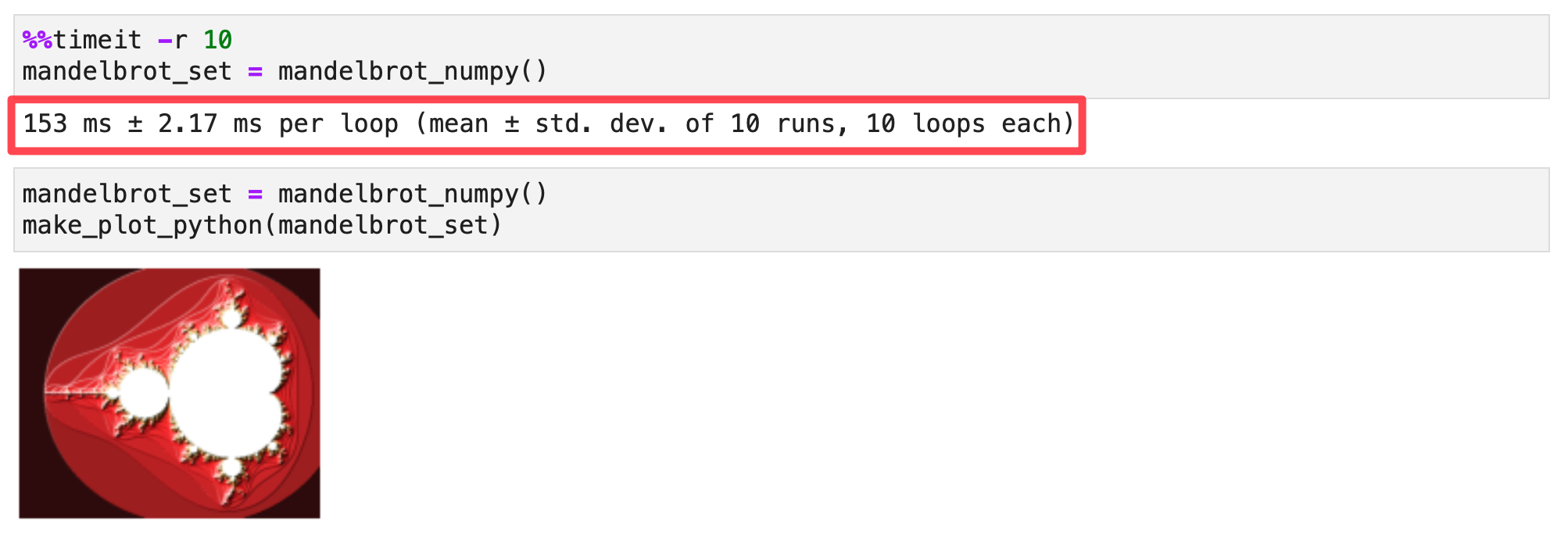## Benchmarking Mojo on the Mojo playground

This comparision is a bit unfair because Mojo🔥 playground has a 32 core Xeon CPU, but I only have a modest 4 year old 8 core desktop CPU. The clock frequency, memory bandwidth and cache sizes and number of cores are all different, but I can’t install PyTorch on Mojo playground so this is the best I can do for comparision for now.

At the bottom of this notebook, I add these few lines of code to measure the execution time of the mandelbrot function.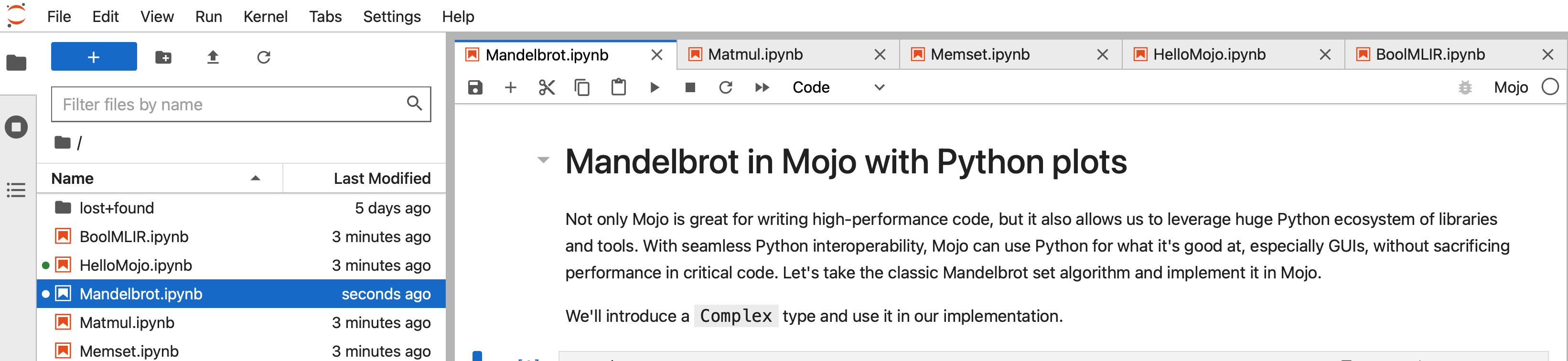``````from Time import now

let eval_begin = now()
let mandelbrot_set = compute_mandelbrot_simd()
let eval_end = now()
let execution_time = (eval_end - eval_begin)

print("execution_time:")
print(F64(execution_time) / 1000000)
``````

Output I found it difficult to benchmark Mojo, because Python benchmarking tools don’t work very readily. I ran the above code several times to ensure that the results are consistent.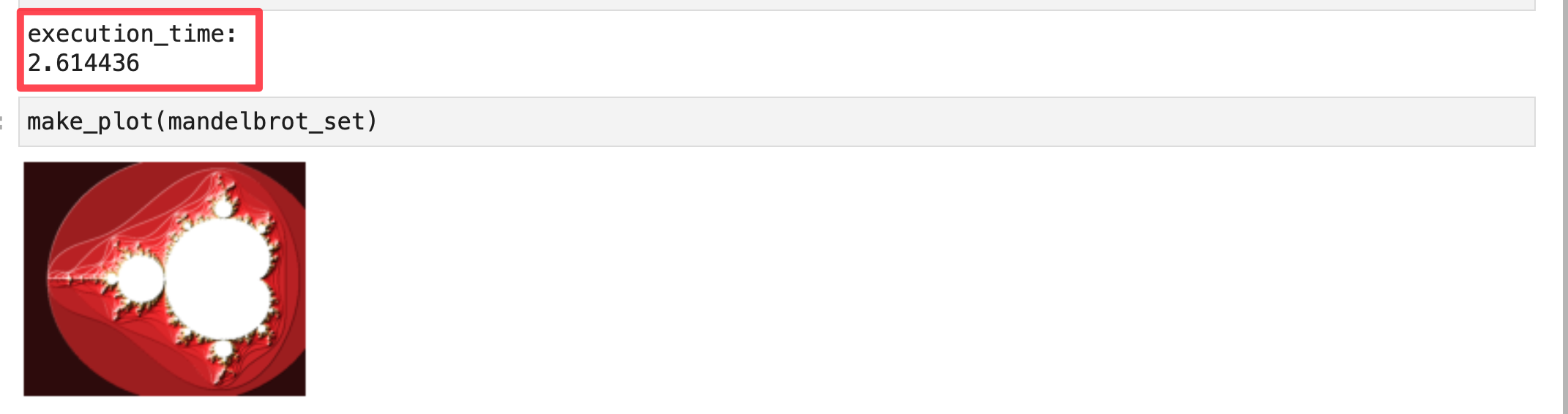## Benchmarking PyTorch CPU

To update our mandelbrot function from numpy implementation to PyTorch implementation I made the following small changes

• replace `np` with `torch`
• add `device=device` to the tensor creation calls, this allows us to pass the appropriate CPU, GPU or Apple MPS device to PyTorch.

Updated mandelbrot function:

``````def mandelbrot_pytorch(device='cpu', max_iter=200):
# Define the boundaries of the complex plane
xn = 450
yn = 375
xmin = -2.25
xmax = 0.75
ymin = -1.25
ymax = 1.25

# Create the grid of complex numbers
x_values = torch.linspace(xmin, xmax, xn, device=device)
y_values = torch.linspace(ymin, ymax, yn, device=device)
rx, iy = torch.meshgrid(x_values, y_values, indexing='xy')

x = rx.clone()
y = iy.clone()
for i in range(max_iter):
x_prev = x
y_prev = y
x = x_prev**2 - y_prev**2 + rx
y = 2*x_prev*y_prev + iy
inside = torch.sqrt(x**2 + y**2) <= 2
``````

Now let’s call our `mandelbrot_pytorch` function with `device='cpu'`## Benchmarking PyTorch CPU with `torch.compile()`

You can compile PyTorch functions using `torch.compile()` and TorchInductor will optimize and generate C++ code with OpenMP pragmas for parallization. This will significantly improve the performance of the function. All of this happens under the hood when you run the code below, but you can pass an additional argument `options={'trace.enabled':True}` to see the generated code. I discuss this is further detail in my PyTorch 2.0 blog post:

##### How Pytorch 2.0 Accelerates Deep Learning with Operator Fusion and CPU/GPU Code-Generation

Let’s compile our mandelbrot function for CPU backend:

``````device = 'cpu'
mandelbrot_compiled = torch.compile(mandelbrot_pytorch)
mandelbrot_set = mandelbrot_compiled(device)
make_plot_python(mandelbrot_set.numpy())
``````

OutputThe generated C++ code looks like this

## Benchmarking PyTorch GPU

``````device = 'cuda'
mandelbrot_set = mandelbrot_pytorch(device)
make_plot_python(mandelbrot_set.cpu().numpy())
``````

Output## Benchmarking PyTorch GPU with `torch.compile()`

``````device = 'cuda'
mandelbrot_compiled = torch.compile(mandelbrot_pytorch)
mandelbrot_set = mandelbrot_compiled(device)
make_plot_python(mandelbrot_set.cpu().numpy())
``````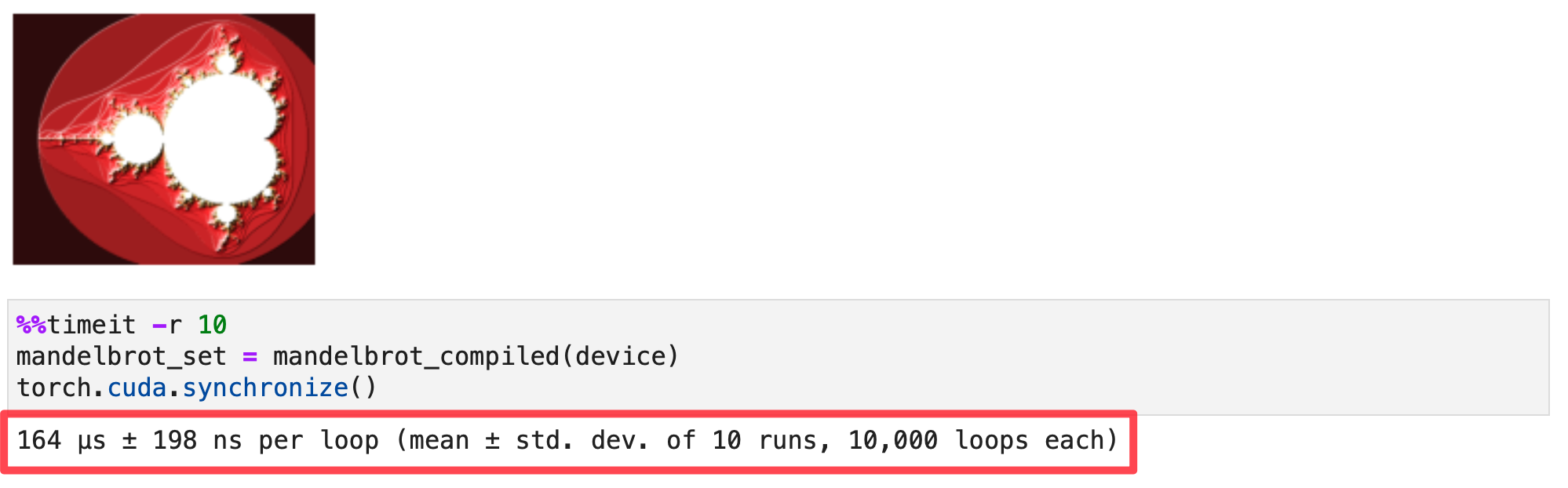The generated OpenAI Triton code which gets compiled to NVPTX for GPUs, looks like this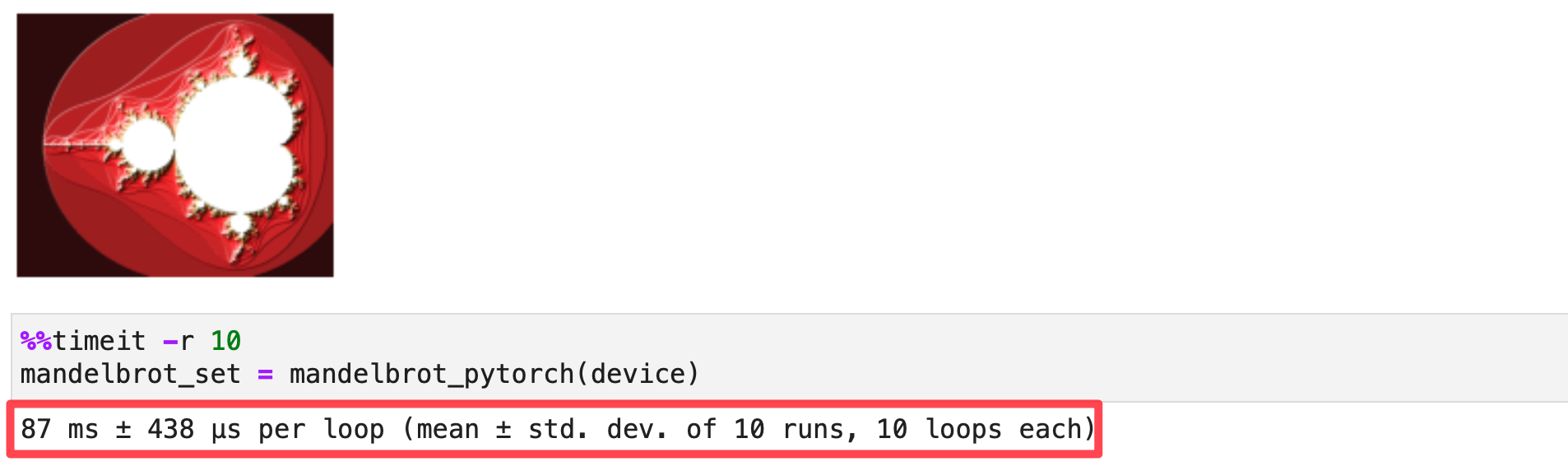That’s it folks! I hope you enjoyed this quick comparision of PyTorch and Mojo🔥. Mojo is fast, but doesn’t have the same level of usability of PyTorch, but that may just be just a matter of time and community support. PyTorch’s one-two punch combo of eager mode with high-level Tensor API and compilation with `torch.compile()` is a powerful combination today. However, the PyTorch ecosystem is also quite fragmented with multiple code paths for different accelerators: TorchInductor (GPUs, CPUs), XLA (TPU, AWS Tranium/Inferentia), custom bindings/bridges (Intel Habana, MPS), and some accelerators like GPUs implement all paths. This leaves the end user and hardware vendors in a dilemma.